# Finally!

Starting Friday, June 11,

## we will be open on Fridays and Saturdays from 10am to 3pm for instore shopping!

Masks are not required, however the team is happy to mask up upon request.
We are excited to have you back in the shop and look forward to your visit!

 table div table+table+table div table{width:100%;padding:0}table div table+table+table div table img{width:96.23%;padding:0;float:none}table div table+table+table div table td{width:100%;padding:0 1.88% 18px}/* styles */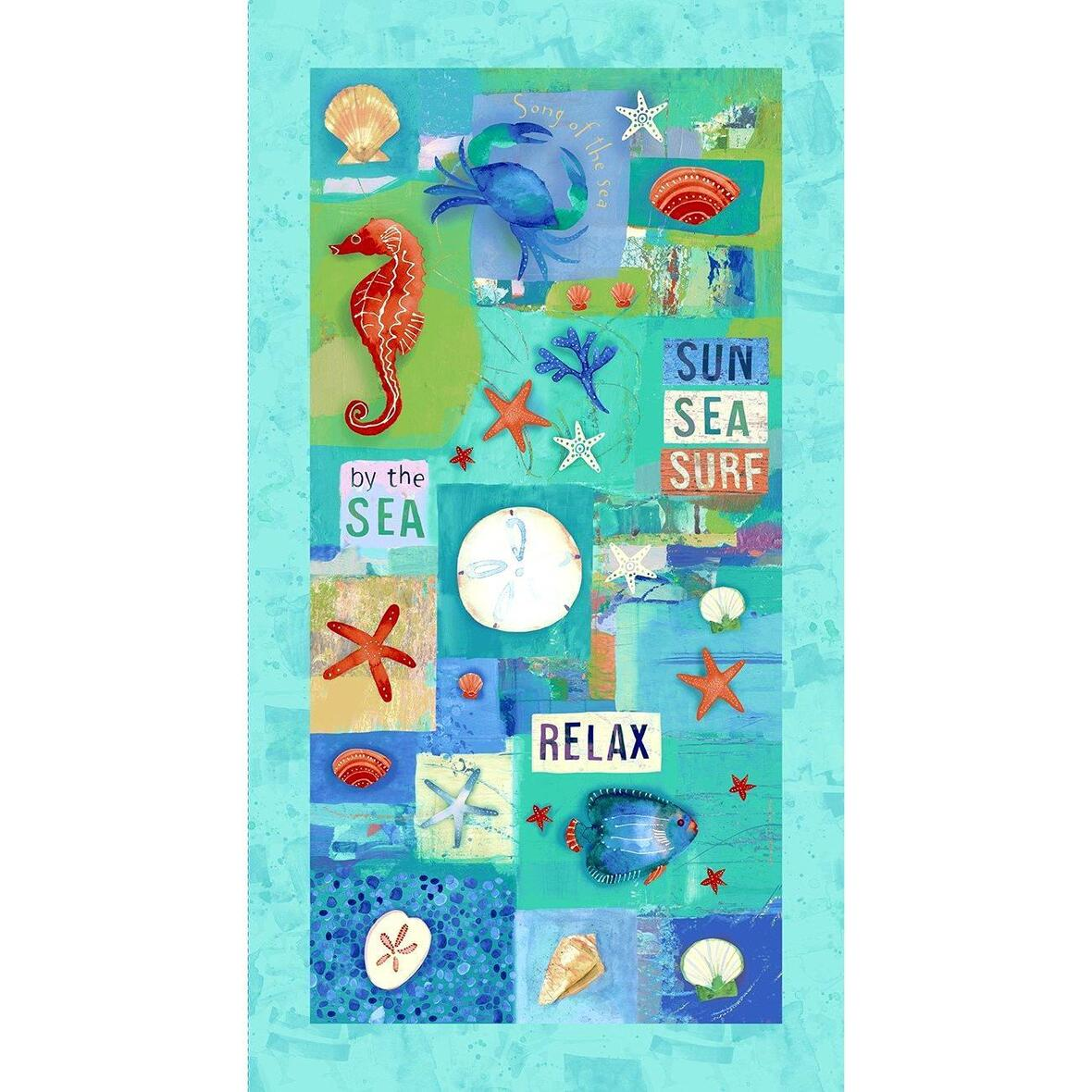# Sanibel

The next best thing to a holiday on Sanibel Island is making something with this beautiful fabric!
Love the colors and the panel is just fun!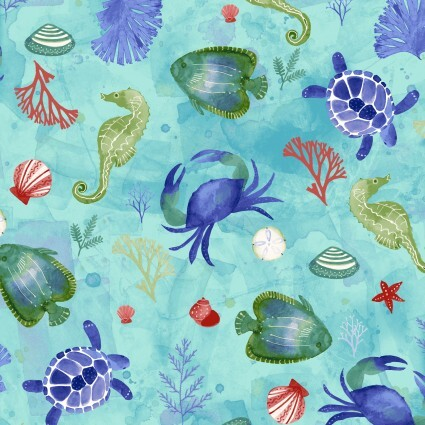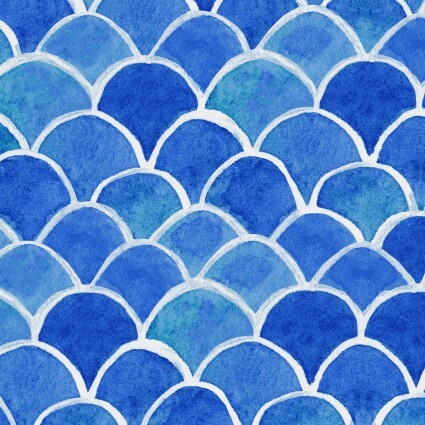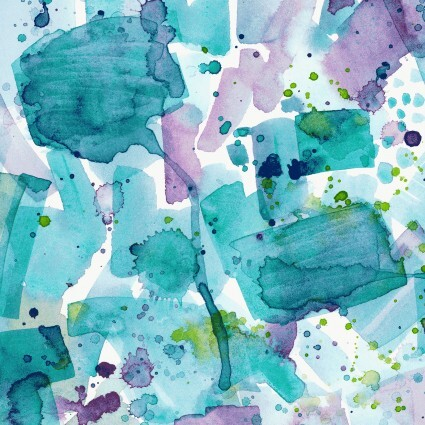table div table+table+table+table+table+table+table div table{width:100%;padding:0}table div table+table+table+table+table+table+table div table img{width:96.23%;padding:0;float:none}table div table+table+table+table+table+table+table div table td{width:100%;padding:0 1.88% 18px}/* styles */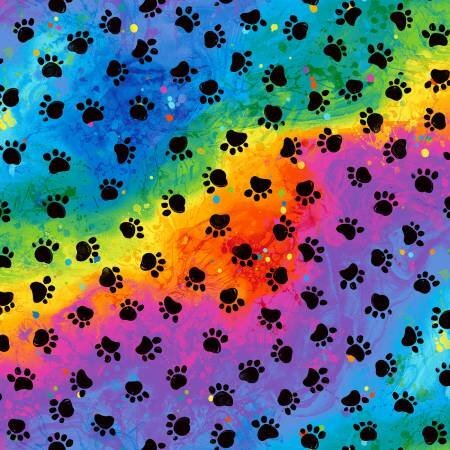# Cats and Dogs and Paws!

Colorful and such fun to look at all the faces and poses of the different cat and dog fabrics we got in, plus some great paw prints too!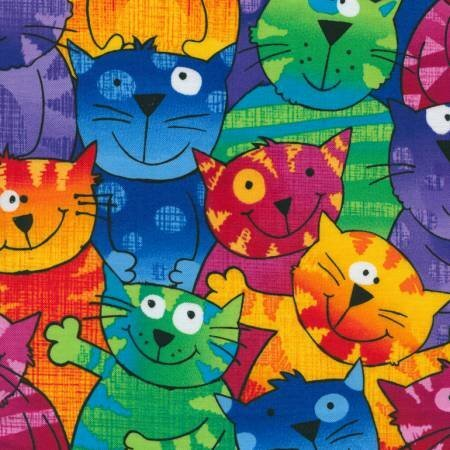table div table+table+table+table+table+table+table+table+table+table+table div table{width:100%;padding:0}table div table+table+table+table+table+table+table+table+table+table+table div table img{width:96.23%;padding:0;float:none}table div table+table+table+table+table+table+table+table+table+table+table div table td{width:100%;padding:0 1.88% 18px}/* styles */# New Patriotic!

Our selection of patriotic fabrics has never been better or bigger!
Fireworks, flags and the Declaration of Independence are new this week!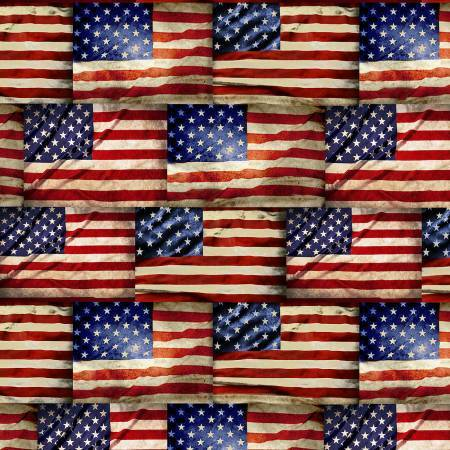table div table+table+table+table+table+table+table+table+table+table+table+table+table+table+table div table{width:100%;padding:0}table div table+table+table+table+table+table+table+table+table+table+table+table+table+table+table div table img{width:96.23%;padding:0;float:none}table div table+table+table+table+table+table+table+table+table+table+table+table+table+table+table div table td{width:100%;padding:0 1.88% 18px}/* styles */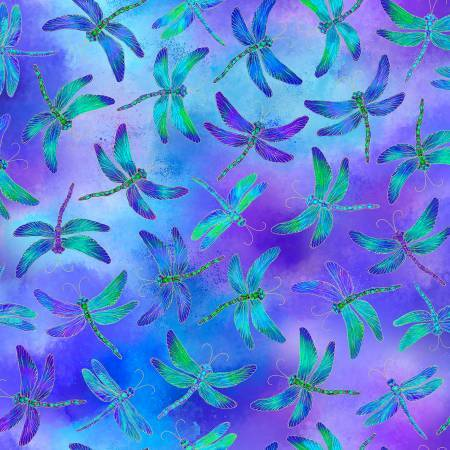# More Great Fabrics!

Floral, golf, horses, dragonflies, gnomes and more - lots of great new fabrics arrived this week!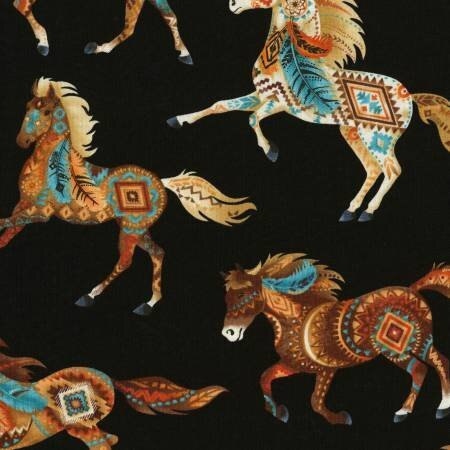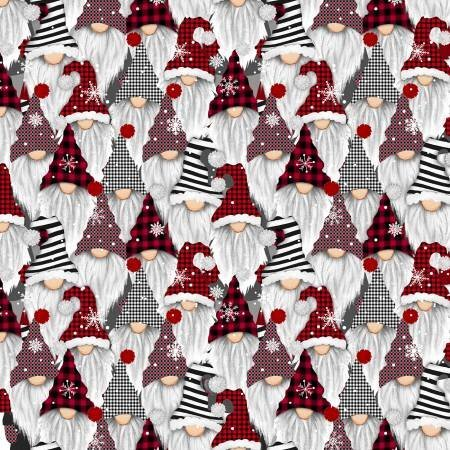table div table+table+table+table+table+table+table+table+table+table+table+table+table+table+table+table+table+table+table div table{width:100%;padding:0}table div table+table+table+table+table+table+table+table+table+table+table+table+table+table+table+table+table+table+table div table img{width:96.23%;padding:0;float:none}table div table+table+table+table+table+table+table+table+table+table+table+table+table+table+table+table+table+table+table div table td{width:100%;padding:0 1.88% 18px}/* styles */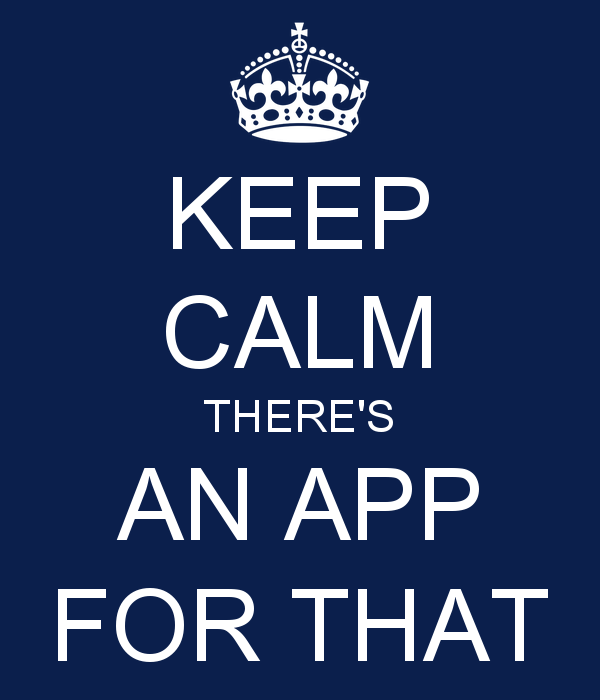# There's an App for that - and we have it!

Cotton Patch now has an app!
It's easy to download
Android users click here
Apple users click here

Why would you want the app?
No need for Facebook - you can watch our FB Live and shop right on the app!
Easy to navigate - there's even a search button!
Shop anytime you like!
App-only sales you won't want to miss!

## Download the app today, you will love it!

 table div table+table+table+table+table+table+table+table+table+table+table+table+table+table+table+table+table+table+table+table+table div table{width:100%;padding:0}table div table+table+table+table+table+table+table+table+table+table+table+table+table+table+table+table+table+table+table+table+table div table img{width:96.23%;padding:0;float:none}table div table+table+table+table+table+table+table+table+table+table+table+table+table+table+table+table+table+table+table+table+table div table td{width:100%;padding:0 1.88% 18px}/* styles */## Be Sure to Catch these Special FB Lives!

 table div table+table+table+table+table+table+table+table+table+table+table+table+table+table+table+table+table+table+table+table+table+table+table div table{width:100%;padding:0}table div table+table+table+table+table+table+table+table+table+table+table+table+table+table+table+table+table+table+table+table+table+table+table div table img{width:96.23%;padding:0;float:none}table div table+table+table+table+table+table+table+table+table+table+table+table+table+table+table+table+table+table+table+table+table+table+table div table td{width:100%;padding:0 1.88% 18px}/* styles */## Sewing Sewcial Encore!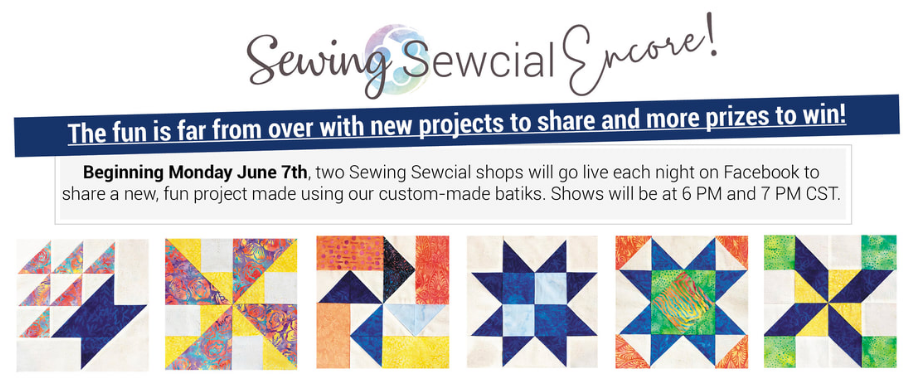/* styles */ ​Monday, June 7th 6 PM - A Scarlet Thread | 7 pm - Prairie Quilt Tuesday, June 8 6 PM - Inspired to Sew | 7 PM - Pine Needles Quilt & Sew Wednesday, June 9 6 PM - Pieceful Quilting | 7 PM - Lone Star Quiltworks Thursday, June 10 6 PM - Cotton Patch Quilt Shop | 7 PM - Off the Rails Quilting Friday, June 11 6 PM - Batiks Etcetera and Sew What Fabrics | 7 PM - Red Pine Quilt Shop Saturday, June 12 6 PM - Calico Gals | 7 PM - Cozy Creative Center (All hours listed are CST.) Each Shop Showcase will take place on their shop Facebook pages. See you there!
 table div table+table+table+table+table+table+table+table+table+table+table+table+table+table+table+table+table+table+table+table+table+table+table+table+table+table+table+table div table{width:100%;padding:0}table div table+table+table+table+table+table+table+table+table+table+table+table+table+table+table+table+table+table+table+table+table+table+table+table+table+table+table+table div table img{width:96.23%;padding:0;float:none}table div table+table+table+table+table+table+table+table+table+table+table+table+table+table+table+table+table+table+table+table+table+table+table+table+table+table+table+table div table td{width:100%;padding:0 1.88% 18px}/* styles */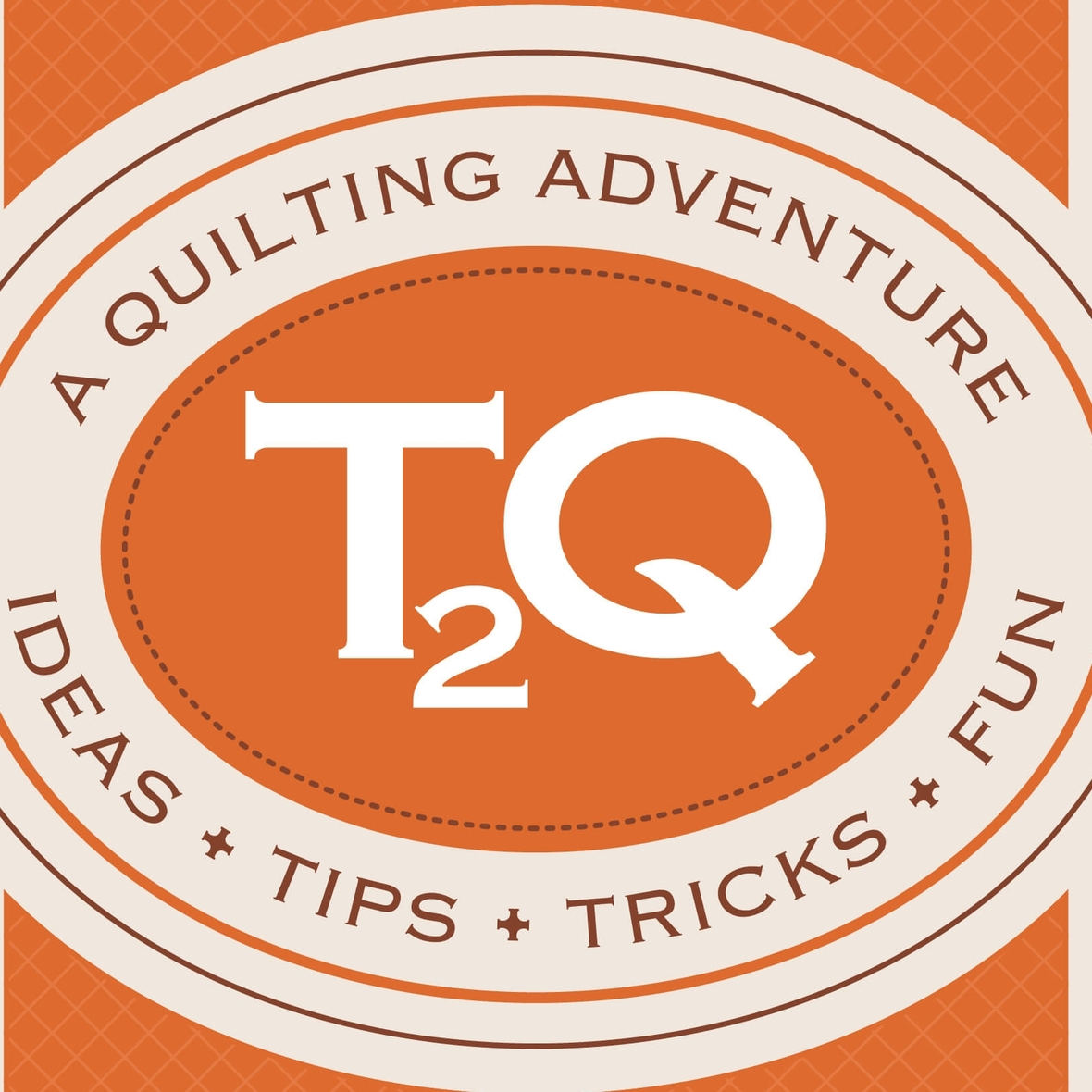# Ticket 2 Quilt!

It's a quilting adventure, it's an online shop hop and Cotton Patch will be in it June 24!
Check out the T2Q Website to see who else is in the June Event!

 table div table+table+table+table+table+table+table+table+table+table+table+table+table+table+table+table+table+table+table+table+table+table+table+table+table+table+table+table+table+table+table div table{width:100%;padding:0}table div table+table+table+table+table+table+table+table+table+table+table+table+table+table+table+table+table+table+table+table+table+table+table+table+table+table+table+table+table+table+table div table img{width:96.23%;padding:0;float:none}table div table+table+table+table+table+table+table+table+table+table+table+table+table+table+table+table+table+table+table+table+table+table+table+table+table+table+table+table+table+table+table div table td{width:100%;padding:0 1.88% 18px}/* styles *//* styles */ Sunday Soiree! FB Live is the place to be Sunday at 7pm! You will be the 1st to see new fabrics, notions, kits and other great things that you need to know about! We put together fun kits that often sell out that very night - you don't want to miss out! There will be prizes, live demos and special pricing! You will be able to purchase during the Live Broadcast and we can ship - sort of like Stuff Your Stocking but all in one night. If you miss the FB Live - not to worry! You can view the replay on our FB Page AND you can still purchase at the special prices until 12pm Monday! Join me for a fun evening!
 table div table+table+table+table+table+table+table+table+table+table+table+table+table+table+table+table+table+table+table+table+table+table+table+table+table+table+table+table+table+table+table+table+table+table+table div table{width:100%;padding:0}table div table+table+table+table+table+table+table+table+table+table+table+table+table+table+table+table+table+table+table+table+table+table+table+table+table+table+table+table+table+table+table+table+table+table+table div table img{width:96.23%;padding:0;float:none}table div table+table+table+table+table+table+table+table+table+table+table+table+table+table+table+table+table+table+table+table+table+table+table+table+table+table+table+table+table+table+table+table+table+table+table div table td{width:100%;padding:0 1.88% 18px}/* styles */# Meet me in the Club House!

Cotton Patch Club House is a new FaceBook group and we want you to be a part of it!
You will find:
An online community for friends of Cotton Patch Quilt Shop!
A place to chat and share photos of your projects.
A place to ask quilty questions, share tips and demos
A place for you to find sneak peeks and early access to items on our FaceBook Live Sunday Soiree!

 table div table+table+table+table+table+table+table+table+table+table+table+table+table+table+table+table+table+table+table+table+table+table+table+table+table+table+table+table+table+table+table+table+table+table+table+table+table+table div table{width:100%;padding:0}table div table+table+table+table+table+table+table+table+table+table+table+table+table+table+table+table+table+table+table+table+table+table+table+table+table+table+table+table+table+table+table+table+table+table+table+table+table+table div table img{width:96.23%;padding:0;float:none}table div table+table+table+table+table+table+table+table+table+table+table+table+table+table+table+table+table+table+table+table+table+table+table+table+table+table+table+table+table+table+table+table+table+table+table+table+table+table div table td{width:100%;padding:0 1.88% 18px}/* styles *//* styles */ Stanley was having a rough day on Sunday, so he hid out underneath the longarm, silly dog!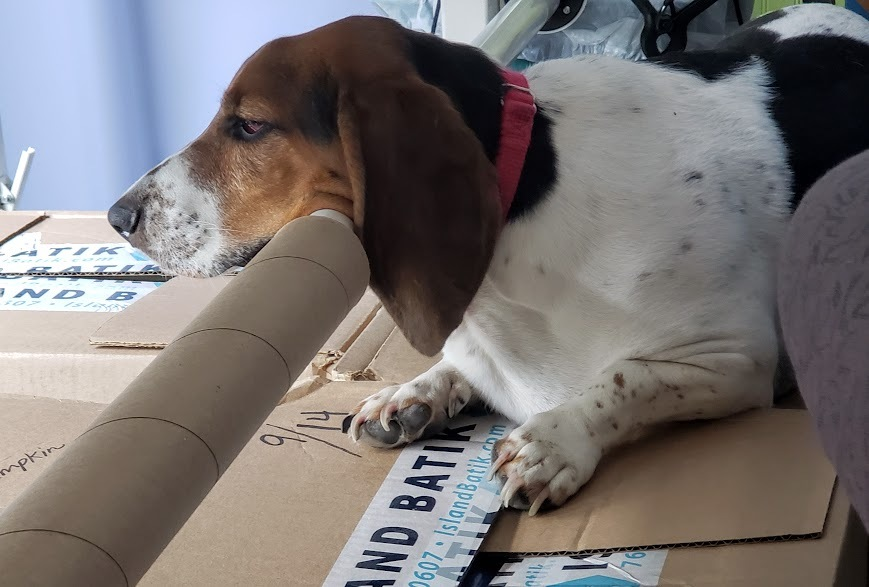Like   Pin   +1